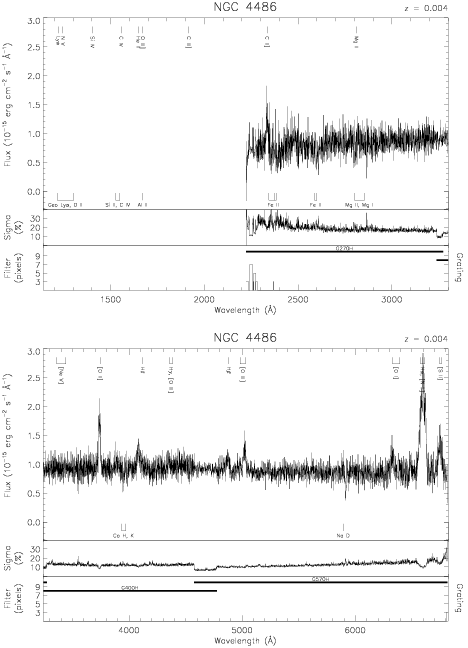Download dataset
TELESCOP= 'HST     '
INSTRUME= 'FOS     '
RADECSYS= 'FK5     '
EQUINOX =               2000.0
DATE-OBS= '1992-12-16T20:26:33'
RA_PNT  =       187.7058604234
DEC_PNT =        12.3910670304
PA_PNT  =        33.9215400000
OBJECT  = 'NGC 4486'
ROOTNM01= 'Y15N0303T'
GRNDMD01= 'SPECTROSCOPY'
DETECT01= 'AMBER   '
APERID01= 'B-2     '
FGWAID01= 'H27     '
ROOTNM02= 'Y15N0304T'
GRNDMD02= 'SPECTROSCOPY'
DETECT02= 'AMBER   '
APERID02= 'B-2     '
FGWAID02= 'H40     '
ROOTNM03= 'Y15N0305T'
GRNDMD03= 'SPECTROSCOPY'
DETECT03= 'AMBER   '
APERID03= 'B-2     '
FGWAID03= 'H57     '